# What is an ideal dipole

## Field lines and equipotential lines of a dipole

The electric field of a dipole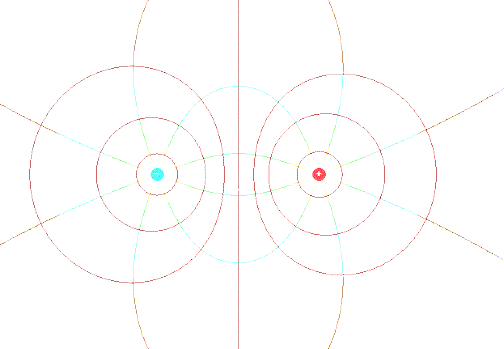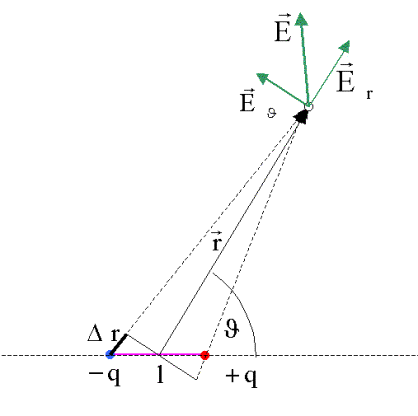We are interested in the electric field generated by the dipole at the starting point with the coordinates r and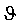. It is assumed that the distance between the dipole charges l is very small compared to r. We calculate the field from the gradient of the potential. The potential results from the summation of the potentials of two point charges: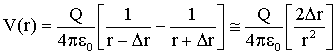Use was made of the fact that Dr2<< r2.

The connection between Dr and can be seen in the last sketchto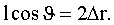This gives the relationship for the potential of the dipole at a sufficient distance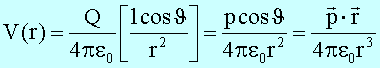The electric field is obtained from the potential by means of the relation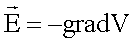.

In spherical coordinates, the gradient is written as follows: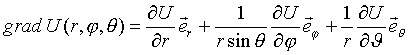Since the problem is rotationally symmetrical with respect to the angle j and therefore V does not depend on j, the electric field is obtained by means of the relationThe radial component of field Er arises tothe azimuth component to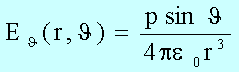The amount of the field strength is obtained with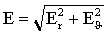to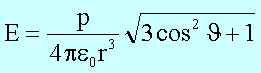The magnitude of the field strength decreases with the third power of the distance from the dipole. In the direction of the dipole axis (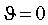) the electric field strength is maximal, the potential is zero. The amount of the electric field strength is minimal perpendicular to the dipole axis. In the direction of the dipole axis, only the radial component contributes to the field, perpendicular to it only the azimuthal component, which because of the rotation by 90 ° also points in the direction of the dipole axis.